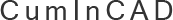CumInCAD is a Cumulative Index about publications in Computer Aided Architectural Design supported by the sibling associations ACADIA, CAADRIA, eCAADe, SIGraDi, ASCAAD and CAAD futures
 PDF papersReferences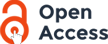id ga0131 Riznyk, V.V. 2001 Perfect Distribution Phenomenon and the Origin of the Spacetime Harmony International Conference on Generative Art Perfect Distribution Phenomenon (PDP), namely one- (t=1) and t-dimensional (t>1) Ideal Ring Relationships (t-D IRR)s are cyclic sequences of integers which form perfect partitions of a finite interval [1,s] of integers. The sums of connected sub-sequences of an IRR enumerate the set of integers [1,s] exactly R-times. Example: The 1-D IRR {1,3,2,7} containing four elements (Fig.1) allows an enumeration of all numbers 1=1, 2=2, 3=3, 4=1+3, 5=3+2, 6=1+3+2, 7=7, ... 13=1+3+2+7 exactly once (R=1). other rvv@polynet.lviv.ua http://www.generativeart.com/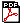file.pdf (91,718 bytes) Content-type: text/plain 2003/08/07 15:25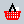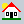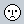CUMINCAD Papers